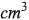9
0
watching
80
views

# The formula for finding the volume of a rectangle is V = lwh, where V is the volume, l is length, w is width, and h is height. If the volume of a rectangle is 108, the length 4 cm, and the width is 3 cm, what is the height of the rectangle?

Get 1 free homework help answer.